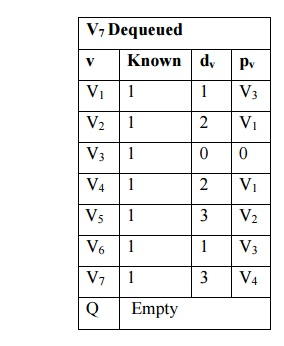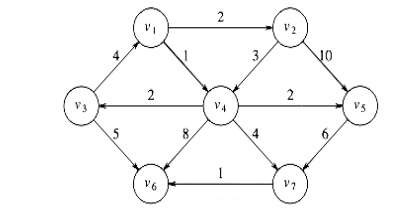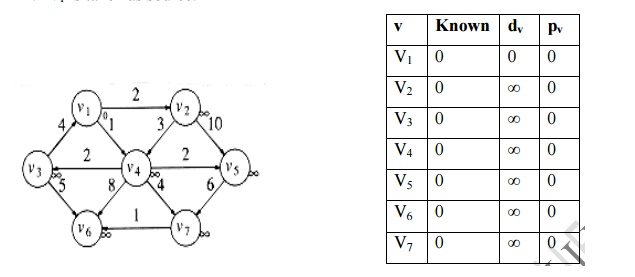Home | | Programming and Data Structure II | DijkstraŌĆÖs Algorithm

# DijkstraŌĆÖs Algorithm

In unweighted shortest path, all the edges are assigned to 1.

DIJKSTRAŌĆÖS ALGORITHM:

Unweighted shortest Path

In unweighted shortest path, all the edges are assigned to 1.

Required Information

i.           known ŌĆō Specifies whether the vertex is processed or not. It is set to 1 after it is processed, otherwise 0. Initially all vertices are unknown, so all entries marked as 0.

ii.           dv ŌĆō it specifies distance form source to vertex. Initially all vertices are unreachable except for S whose path length is zero.

iii.           pv ŌĆō It is book keeping variable, which allow us to print te actual path. i.e., the vertex which makes the changes in dv.

Procedure to find the unweighted shortest path

i.           Assign the source node as S and Enqueue S.

ii.           Dequeue the vertex S from queue and assign the value of that vertex to be known and then find its adjacency vertices.

iii.           If the distance of the adjacent vertices is equal to infinity then change the distance of that vertex as the distance of its source vertex. Increment by 1 and enqueue the vertex.

iv.          Repeat step ii until the queue becomes empty.

Routine for unweighted shortest path

void unweighted( Table T )

{

Queue Q; Vertex v, w;

Q = CreateQueue( NumVertex );

MakeEmpty( Q );

Enqueue( S, Q ); while( !IsEmpty( Q ) )

{

v = Dequeue( Q );

T[v].known = True;

for each w adjacent to v

if( T[w].Dist = = INFINITY )

{

T[w].Dist = T[v].Dist + 1;

T[w].path = v;

Enqueue( w, Q );

}}

DisposeQueue( Q ); /* free the memory */

}

Examplei.                   v3 is taken as source node and its path length is initialized to 0. v3 is inserted into Q.ii.           v3 find its adjacent node whose path length is 1. v1, v6 are adjacent nodes to v3 and inserted in to queue.iii.           Find the adjacent node for v1. v2 and v4 are adjacent node for v1, v2 and v4 inserted into the queue.iv.          No adjacent vertices for v6. No change in path value for all vertices.v.           Find the adjacent vertices for v2. v4 and v5 are adjacent nodes to v2 and inserted into queue.vi.          Find the adjacent vertices for v4. v5, v6 and v7 are adjacent vertices. Minimum path length for v7 is 3. v7 is inserted into queue.vii.          An adjacent vertex for v5 is v7. Already found the minimum path length from v3 to v7 is 3. So no change in dv and pv.viii.          An adjacent vertex for v7 is v6. Already found the minimum path length from v3 to v6 is 1. So no change in dv and pv.Algorithm Analysis

Running time is O(|E| + |V|)

Weighted Graph

The general method to solve the single source shortest path problem is known as

DijkstraŌĆ¤s algorithm. It applied to weighted graph.

Procedure

├ś   It uses greedy technique.

├ś   It proceeds in stages.

├ś   It selects a vertex v, which has the smallest dv among all the unknown vertices and declares the shortest path from s to v is known.

├ś   The remainder consists of updating the value of dw.

├ś   We should  dw = dv + Cv, w, if the new value for dw would an improvement.

Example: Find the shortest path for the following graph.1.       v1 is taken as source.2.     Now v1 is known vertex, marked as 1. Its adjacent vertices are v2, v4, pv and dv values are updated

T[v2]. dist = Min (T[v2].dist, T[v1].dist + Cv1, v2) = Min (╬▒ , 0+2) = 2

T[v4]. dist = Min (T[v4].dist, T[v1].dist + Cv1, v4) = Min (╬▒ , 0+1) = 13.  Select the vertex with minimum distance away v2 and v4 . v4 is marked as known vertex. Its adjacent vertices are v3, v5, v6 and v7 .

T[v3]. dist = Min (T[v3].dist, T[v4].dist + Cv4, v3) = Min (╬▒ , 1+2) = 3

T[v5]. dist = Min (T[v5].dist, T[v4].dist + Cv4, v5) = Min (╬▒ , 1+2) = 3

T[v6]. dist = Min (T[v6].dist, T[v4].dist + Cv4, v6) = Min (╬▒ , 1+8) = 9

T[v7]. dist = Min (T[v7].dist, T[v4].dist + Cv4, v7) = Min (╬▒ , 1+4) = 54.     Select the vertex which is shortest distance from source v1. v2 is smallest one. v2 is marked as known vertex. Its adjacent vertices are v4 ad v5. The distance from v1 to vand v5 through v2 is more comparing with previous value of dv. No change in dv and pv value.5.     Select tht smallest vertex from source. v3 and v5 are smallest one. Adjacent vertices for v3 is v1 and v6. v1 is source there is no change in dv and pv

T[v6]. dist = Min (T[v6].dist, T[v3].dist + Cv3, v6) = Min (9 , 3+5) = 8

dv and pv values are updated. Adjacent vertices for v5 is v7. No change in dv and pv value.6. Next smallest vertex v7. Its adjacent vertex is v6. T[v6]. dist = Min (T[v6].dist, T[v7].dist + Cv7, v6) = Min (8 , 5+1) = 6

dv and pv values are updated.7. The last vertex v6 is declared as known. No adjacent vertices for v6. No updation in the table.The shortest distance from source v1 to all vertices. v1 -> v2 = 2

v1 -> v3 = 3 v1 -> v4 = 1 v1 -> v5 = 3 v1 -> v6 = 6 v1 -> v7 = 5

Algorithm Analysis

Time complexity of this algorithm

O(|E| + |V|2 ) = O(|V|2 )

Declarations for DijkstraŌĆÖs algorithm

typedef int Vertex; struct TableEntry

{

int known;

DistType Dist;

Vertex Path

};

/* Vertices are numbered from 0*/ #define NotAVertex (-1)

typedef struct TableEntry Table[NumVertex];

Table Initialization routine

void InitTable(Vertex Start, Graph G, Table T)

{

for (i=0; i<NumVertex; i++)

{

T[i].known = False;

T[i]. Dist = Infinity;

T[i]. Path = NotAVertex;

}

T[Start]. Dist = 0;

}

Routine to print the actual shortest path

void Printpath(Vertex V, Table T)

{

if(T[V]. Path != NotAVertex)

{

PrintPath(T[V]. Path, T); printf(ŌĆ£toŌĆØ);

}

printf(ŌĆ£%vŌĆØ, V);

/* %v is pseudocode*/

}

Pseudocode for DijkstraŌĆÖs algorithm

void Dijkstra(Table T)

{

Vertex v, w; for( ; ;)

{

v = smallest unknown distance vertex;

if( v = = NotAVertex)

break;

T[v]. kown = True;

for each w adjacent to v if(!T[w].known)

if(T[v].Dist + Cvw < T[w]. Dist)

{

/* update w*/

Decrease(T[w]. Dist to T[v].Dist + Cvw);

T[w]. path = v;

}

}

}

Study Material, Lecturing Notes, Assignment, Reference, Wiki description explanation, brief detail
Programming and Data structures : Graphs : DijkstraŌĆÖs Algorithm |